# Feedback Control Systems FCS Lecture10 11 Mathematical Modelling

• Slides: 46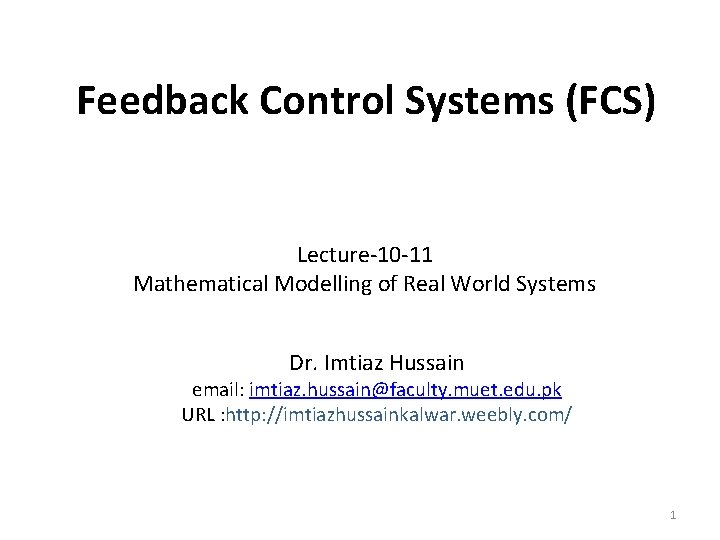Feedback Control Systems (FCS) Lecture-10 -11 Mathematical Modelling of Real World Systems Dr. Imtiaz Hussain email: imtiaz. [email protected] muet. edu. pk URL : http: //imtiazhussainkalwar. weebly. com/ 1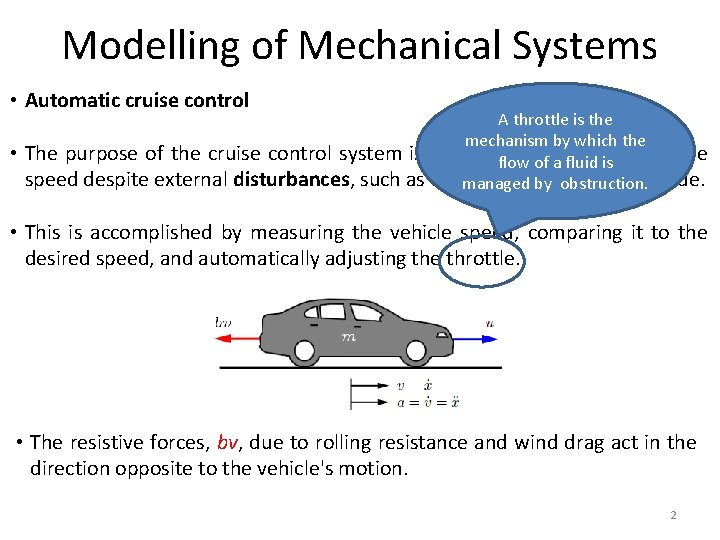Modelling of Mechanical Systems • Automatic cruise control • A throttle is the mechanism by which the The purpose of the cruise control system is to maintain a constant vehicle flow of a fluid is speed despite external disturbances, such as changes in wind or road grade. managed by obstruction. • This is accomplished by measuring the vehicle speed, comparing it to the desired speed, and automatically adjusting the throttle. • The resistive forces, bv, due to rolling resistance and wind drag act in the direction opposite to the vehicle's motion. 2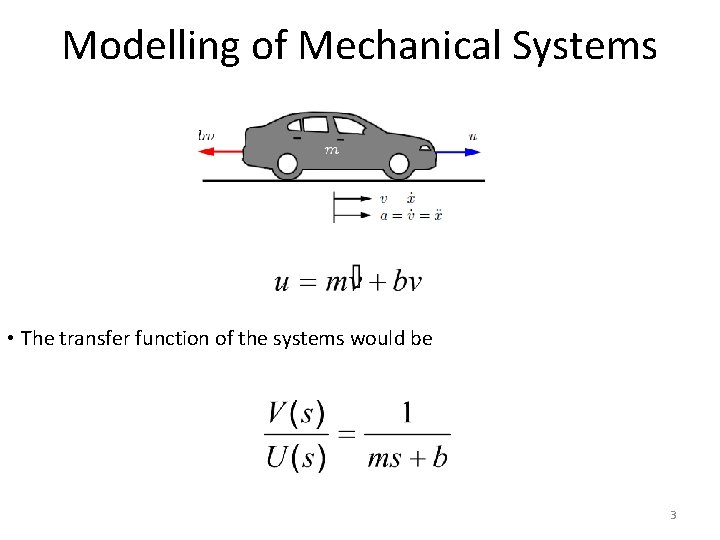Modelling of Mechanical Systems • The transfer function of the systems would be 3Modelling of Mechanical Systems • Consider a simple pendulum shown below. 4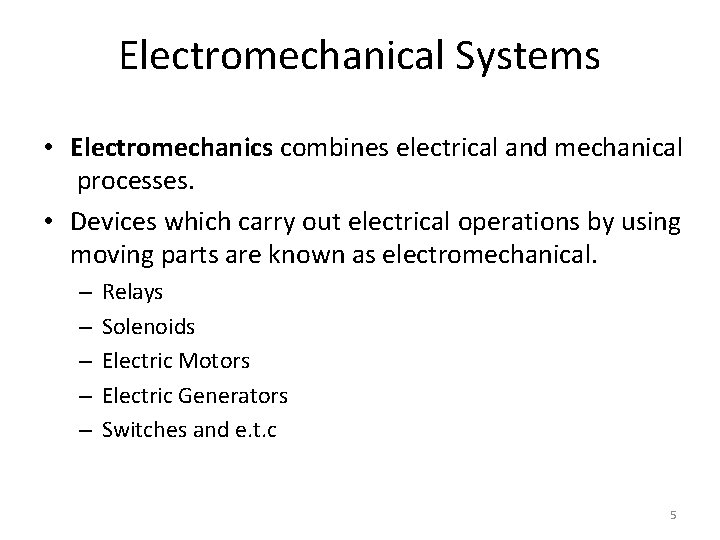Electromechanical Systems • Electromechanics combines electrical and mechanical processes. • Devices which carry out electrical operations by using moving parts are known as electromechanical. – – – Relays Solenoids Electric Motors Electric Generators Switches and e. t. c 5Introduction • Micro-electromechanical (MEMS) systems is the technology of very small devices. • It merges at the nano-scale into nanoelectromechanical systems(NEMS) and nanotechnology. 6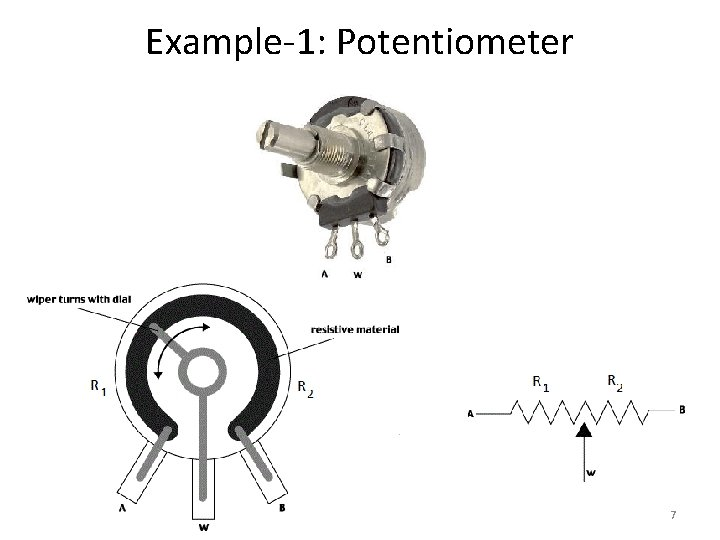Example-1: Potentiometer 7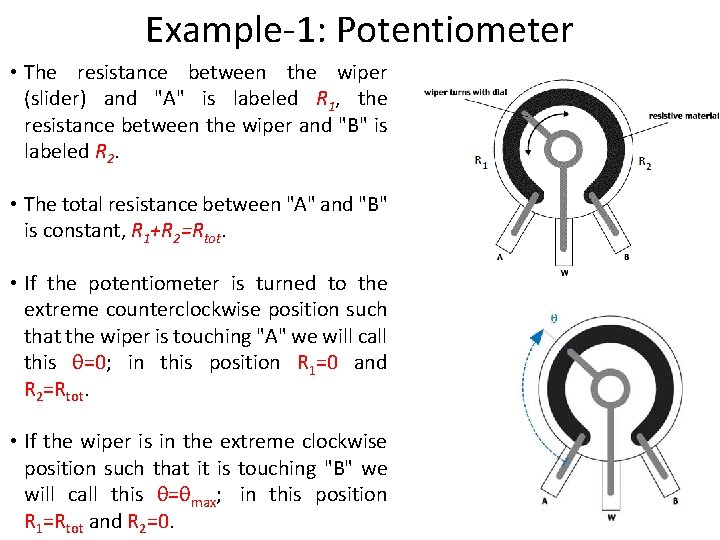Example-1: Potentiometer • The resistance between the wiper (slider) and "A" is labeled R 1, the resistance between the wiper and "B" is labeled R 2. • The total resistance between "A" and "B" is constant, R 1+R 2=Rtot. • If the potentiometer is turned to the extreme counterclockwise position such that the wiper is touching "A" we will call this θ=0; in this position R 1=0 and R 2=Rtot. • If the wiper is in the extreme clockwise position such that it is touching "B" we will call this θ=θmax; in this position R 1=Rtot and R 2=0. 8Example-1: Potentiometer • R 1 and R 2 vary linearly with θ between the two extremes: 9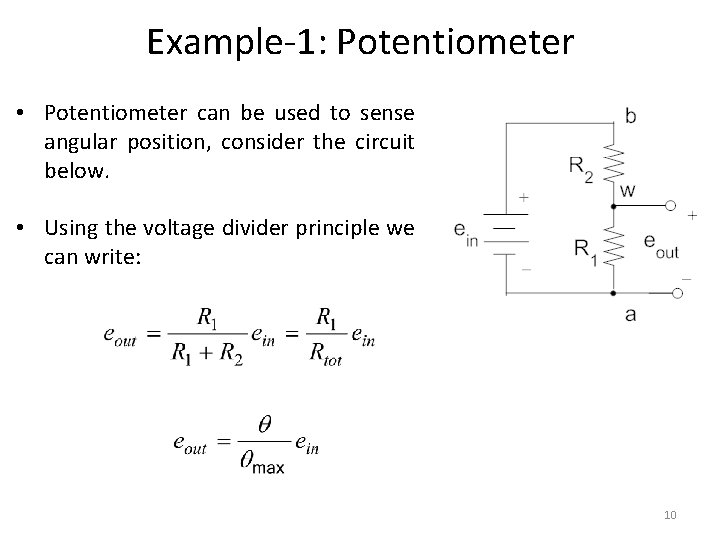Example-1: Potentiometer • Potentiometer can be used to sense angular position, consider the circuit below. • Using the voltage divider principle we can write: 10Example-2: Loud Speaker • A voltage is typically applied across the terminals of the loudspeaker and the "cone" moves in and out causing pressure waves perceived as sound. 11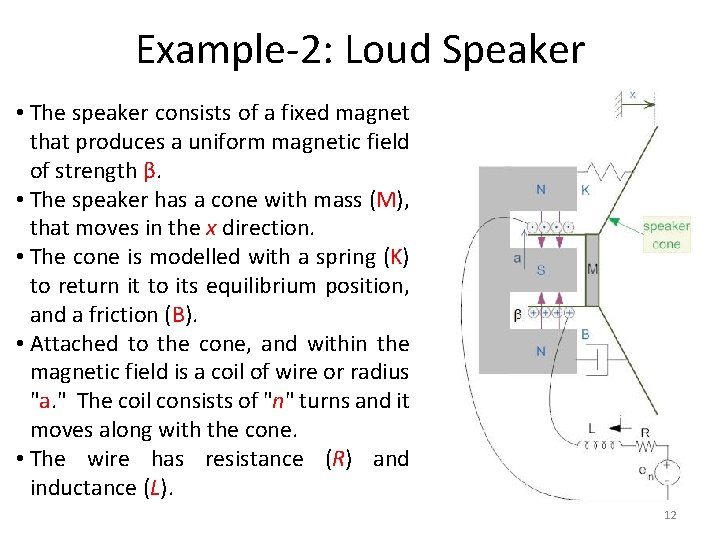Example-2: Loud Speaker • The speaker consists of a fixed magnet that produces a uniform magnetic field of strength β. • The speaker has a cone with mass (M), that moves in the x direction. • The cone is modelled with a spring (K) to return it to its equilibrium position, and a friction (B). • Attached to the cone, and within the magnetic field is a coil of wire or radius "a. " The coil consists of "n" turns and it moves along with the cone. • The wire has resistance (R) and inductance (L). 12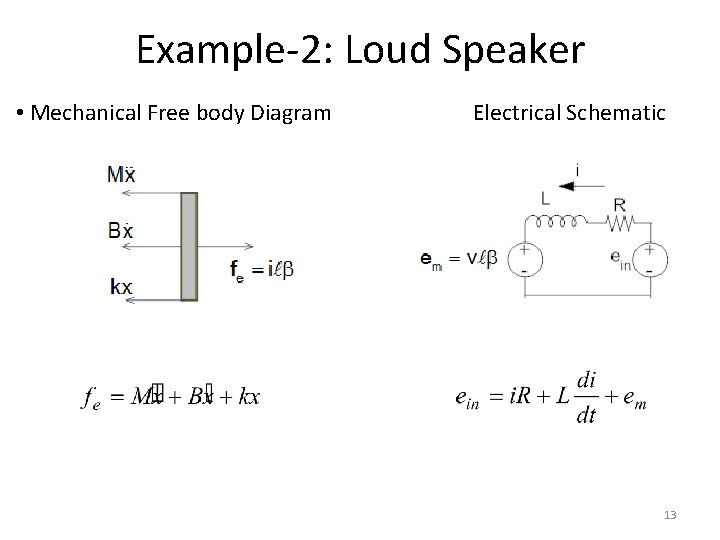Example-2: Loud Speaker • Mechanical Free body Diagram Electrical Schematic 13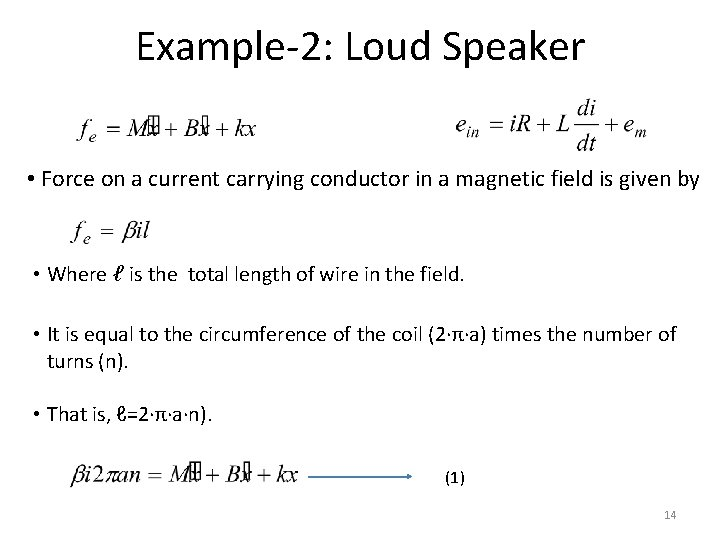Example-2: Loud Speaker • Force on a current carrying conductor in a magnetic field is given by • Where ℓ is the total length of wire in the field. • It is equal to the circumference of the coil (2·π·a) times the number of turns (n). • That is, ℓ=2·π·a·n). (1) 14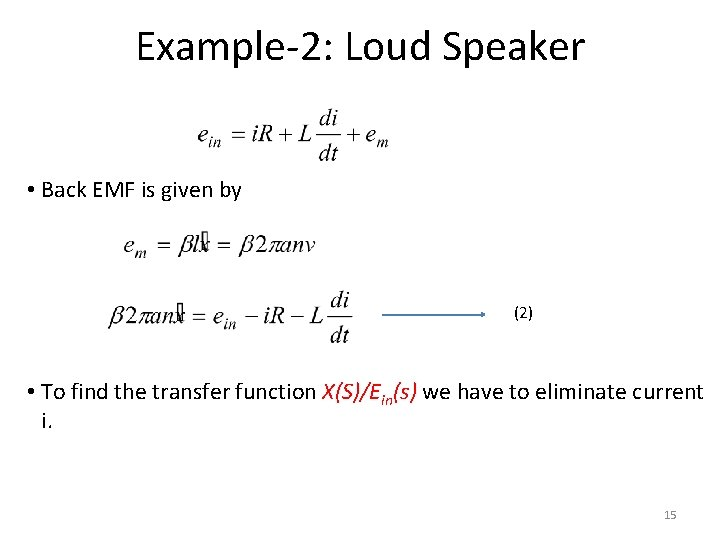Example-2: Loud Speaker • Back EMF is given by (2) • To find the transfer function X(S)/Ein(s) we have to eliminate current i. 15Example-2: Loud Speaker (2) (1) • Taking Laplace transform of equations (1) and (2) considering initial conditions to zero. (3) (4) • Re-arranging equation (3) as 16Example-2: Loud Speaker • Put I(s) in equation (4) • After simplification the transfer function is calculated as 17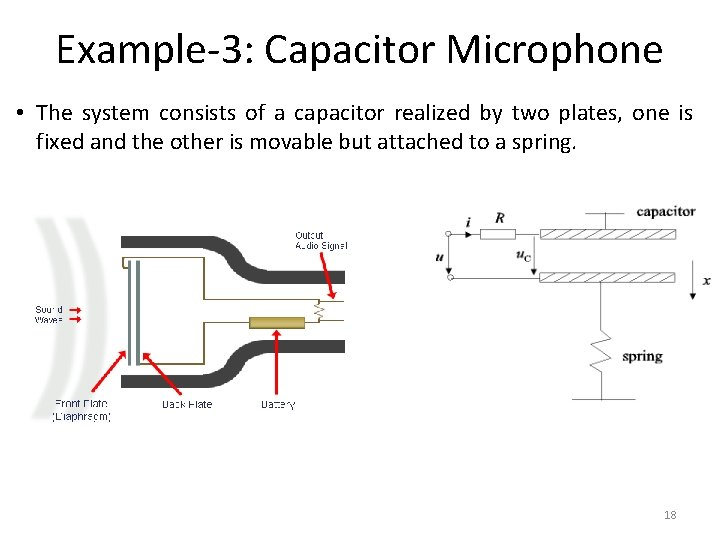Example-3: Capacitor Microphone • The system consists of a capacitor realized by two plates, one is fixed and the other is movable but attached to a spring. 18Example-4: Electromagnetic Relay 19Example-5: Push Button 20Example-6: D. C Drives • Speed control can be achieved using DC drives in a number of ways. • Variable Voltage can be applied to the armature terminals of the DC motor. • Another method is to vary the flux per pole of the motor. • The first method involve adjusting the motor’s armature while the latter method involves adjusting the motor field. These methods are referred to as “armature control” and “field control. ” 21Example-6: D. C Drives • Motor Characteristics • For every motor, there is a specific Torque/Speed curve and Power curve. • Torque is inversely proportional to the speed of the output shaft. • Motor characteristics are frequently given as two points on this graph: • The stall torque, , represents the point on the graph at which the torque is maximum, but the shaft is not rotating. • The no load speed is the maximum output speed of the motor. 22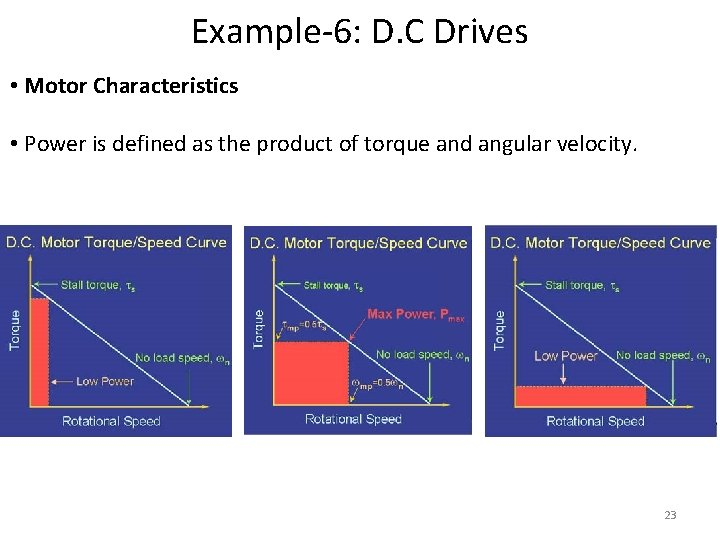Example-6: D. C Drives • Motor Characteristics • Power is defined as the product of torque and angular velocity. 23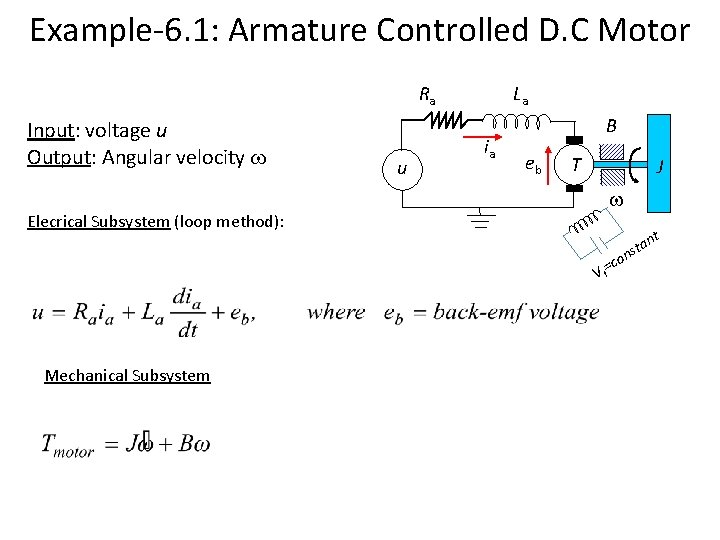Example-6. 1: Armature Controlled D. C Motor Ra Input: voltage u Output: Angular velocity u La ia B eb T J Elecrical Subsystem (loop method): nt sta n =co Vf Mechanical SubsystemExample-6. 1: Armature Controlled D. C Motor Ra Power Transformation: u La ia B eb T J Torque-Current: Voltage-Speed: where Kt: torque constant, Kb: velocity constant For an ideal motor Combing previous equations results in the following mathematical model: t an t s n =co VfExample-6. 1: Armature Controlled D. C Motor Taking Laplace transform of the system’s differential equations with zero initial conditions gives: Eliminating Ia yields the input-output transfer function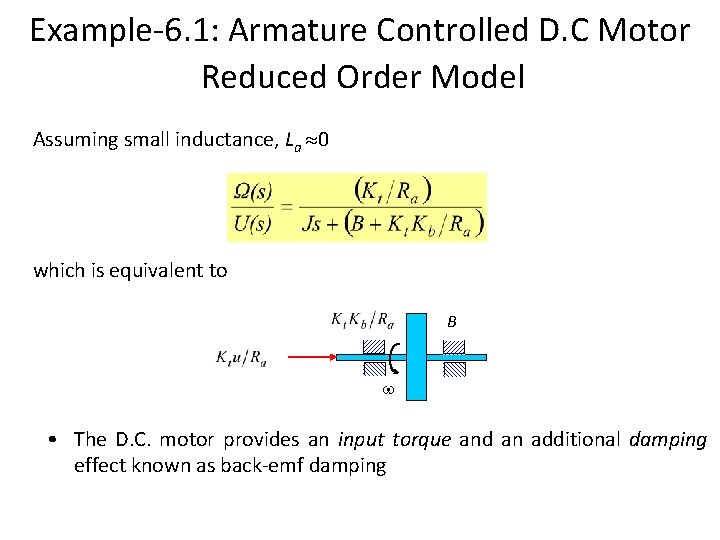Example-6. 1: Armature Controlled D. C Motor Reduced Order Model Assuming small inductance, La 0 which is equivalent to B • The D. C. motor provides an input torque and an additional damping effect known as back-emf damping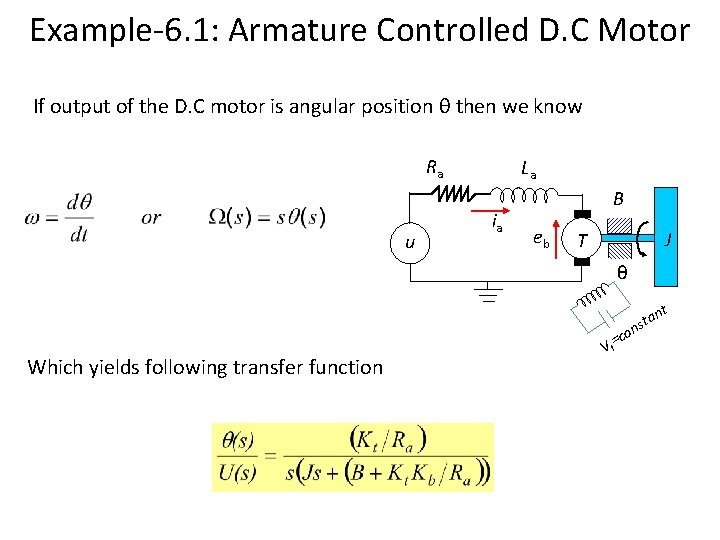Example-6. 1: Armature Controlled D. C Motor If output of the D. C motor is angular position θ then we know Ra u La ia B eb J T θ t an t s n =co Which yields following transfer function Vf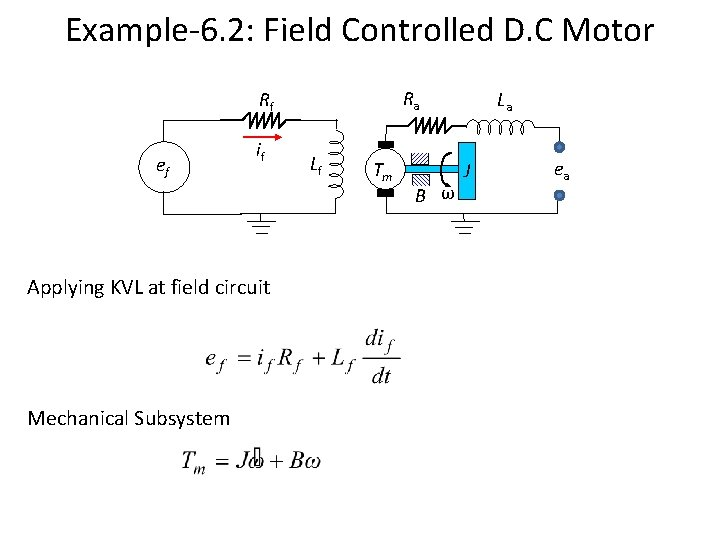Example-6. 2: Field Controlled D. C Motor Ra Rf ef if Applying KVL at field circuit Mechanical Subsystem Lf Tm B ω La J ea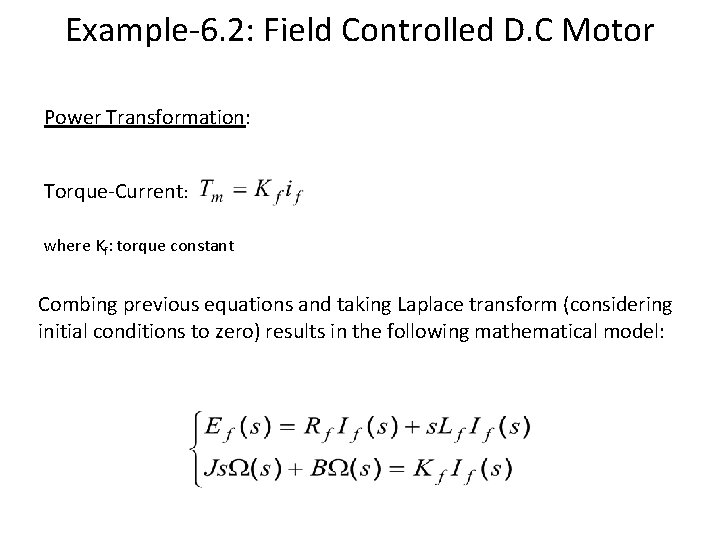Example-6. 2: Field Controlled D. C Motor Power Transformation: Torque-Current: where Kf: torque constant Combing previous equations and taking Laplace transform (considering initial conditions to zero) results in the following mathematical model:Example-6. 2: Field Controlled D. C Motor Eliminating If(S) yields If angular position θ is output of the motor Ra Rf ef if Lf Tm La J B θ eaExample-6. 3 An armature controlled D. C motor runs at 5000 rpm when 15 v applied at the armature circuit. Armature resistance of the motor is 0. 2 Ω, armature inductance is negligible, back emf constant is 5. 5 x 10 -2 v sec/rad, motor torque constant is 6 x 10 -5, moment of inertia of motor 10 -5, viscous friction coeffcient is negligible, moment of inertia of load is 4. 4 x 10 -3, viscous friction coeffcient of load is 4 x 10 -2. La Ra ea 15 v ia N 1 Bm eb T Jm BL t =co an t s n Vf JL N 2 L 1. Drive the overall transfer function of the system i. e. ΩL(s)/ Ea(s) 2. Determine the gear ratio such that the rotational speed of the load is reduced to half and torque is doubled.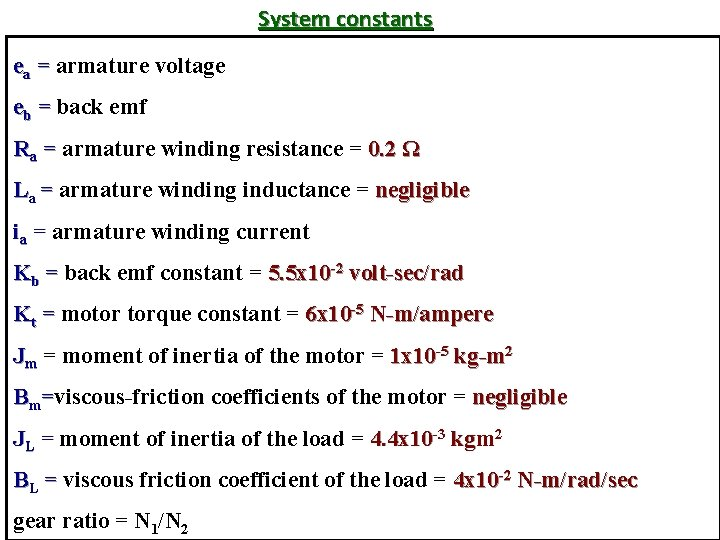System constants ea = armature voltage eb = back emf Ra = armature winding resistance = 0. 2 Ω La = armature winding inductance = negligible ia = armature winding current Kb = back emf constant = 5. 5 x 10 -2 volt-sec/rad Kt = motor torque constant = 6 x 10 -5 N-m/ampere Jm = moment of inertia of the motor = 1 x 10 -5 kg-m 2 Bm=viscous-friction coefficients of the motor = negligible JL = moment of inertia of the load = 4. 4 x 10 -3 kgm 2 BL = viscous friction coefficient of the load = 4 x 10 -2 N-m/rad/sec gear ratio = N 1/N 2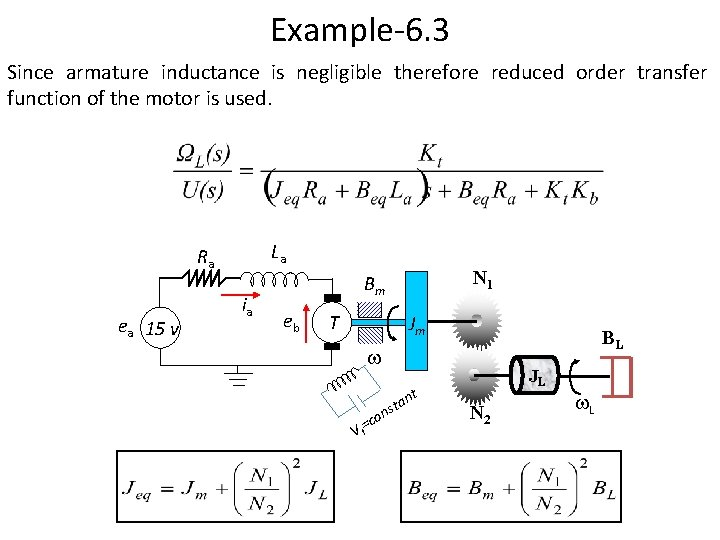Example-6. 3 Since armature inductance is negligible therefore reduced order transfer function of the motor is used. La Ra ea 15 v ia N 1 Bm eb T Jm BL t =co an t s n Vf JL N 2 LExample-6. 4 A field controlled D. C motor runs at 10000 rpm when 15 v applied at the field circuit. Filed resistance of the motor is 0. 25 Ω, Filed inductance is 0. 1 H, motor torque constant is 1 x 10 -4, moment of inertia of motor 10 -5, viscous friction coefficient is 0. 003, moment of inertia of load is 4. 4 x 10 -3, viscous friction coefficient of load is 4 x 10 -2. Ra Rf ef if Lf Tm La ea B m ωm N 1 Jm BL JL N 2 L 1. Drive the overall transfer function of the system i. e. ΩL(s)/ Ef(s) 2. Determine the gear ratio such that the rotational speed of the load is reduced to 500 rpm.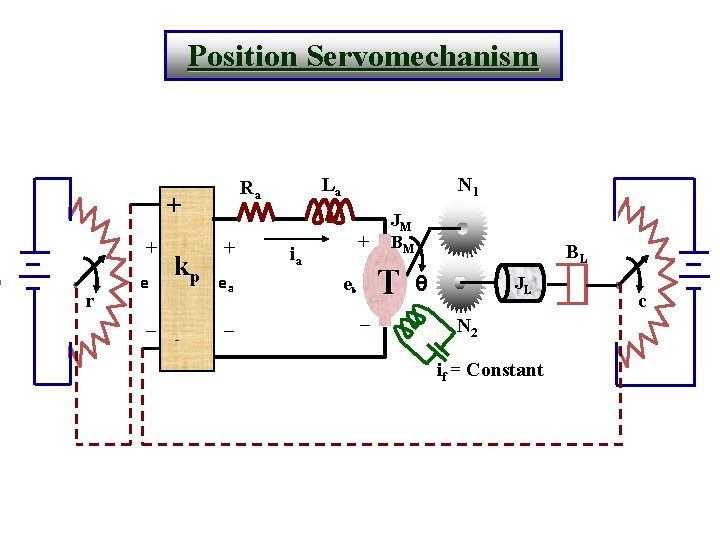Position Servomechanism + + r e _ kp - La Ra + ea _ N 1 + ia JM BM T e b _ BL θ JL N 2 if = Constant c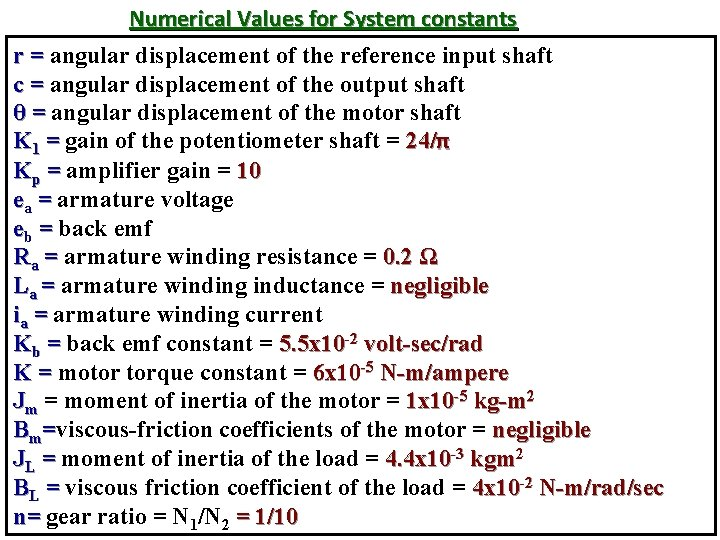Numerical Values for System constants r = angular displacement of the reference input shaft c = angular displacement of the output shaft θ = angular displacement of the motor shaft K 1 = gain of the potentiometer shaft = 24/π Kp = amplifier gain = 10 ea = armature voltage eb = back emf Ra = armature winding resistance = 0. 2 Ω La = armature winding inductance = negligible ia = armature winding current Kb = back emf constant = 5. 5 x 10 -2 volt-sec/rad K = motor torque constant = 6 x 10 -5 N-m/ampere Jm = moment of inertia of the motor = 1 x 10 -5 kg-m 2 Bm=viscous-friction coefficients of the motor = negligible JL = moment of inertia of the load = 4. 4 x 10 -3 kgm 2 BL = viscous friction coefficient of the load = 4 x 10 -2 N-m/rad/sec n= gear ratio = N 1/N 2 = 1/10System Equations e(t)=K 1[ r(t) - c(t) ] or E(S)=K 1 [ R(S) - C(S) ] Ea(s)=Kp E(S) (1) (2) Transfer function of the armature controlled D. C motor Is given by Km = Ea(S) S(Tm. S+1) θ(S)System Equations (contd…. . ) Where K Km = Ra. Beq+KKb And Tm Also Ra. Jeq = Ra. Beq+KKb Jeq=Jm+(N 1/N 2)2 JL Beq=Bm+(N 1/N 2)2 BL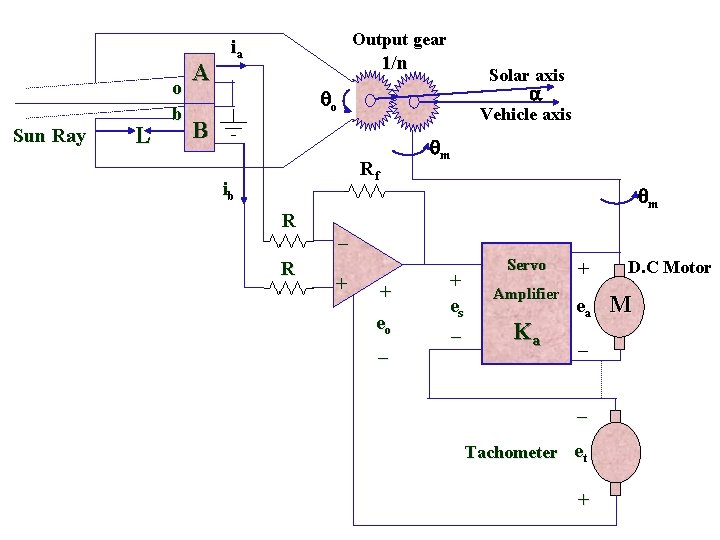Sun Ray L o b A Output gear ia 1/n Solar axis o Vehicle axis B m Rf ib R R m _ + + eo _ + es _ Servo Amplifier Ka + ea _ _ Tachometer et + D. C Motor M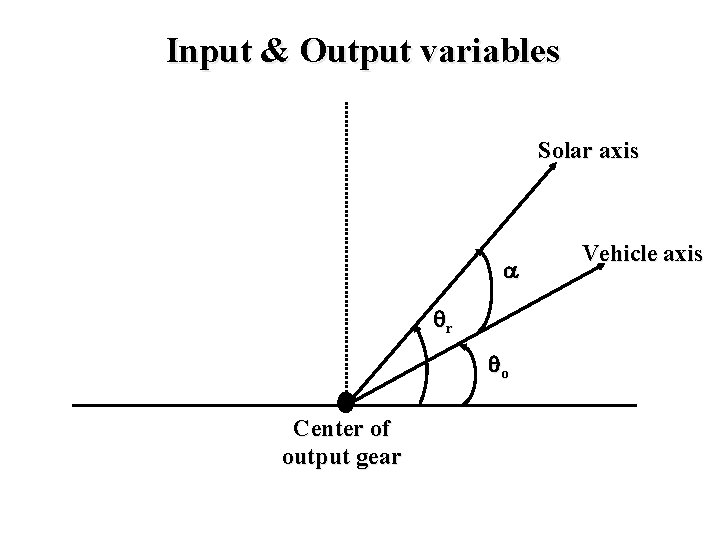Input & Output variables Solar axis r o Center of output gear Vehicle axis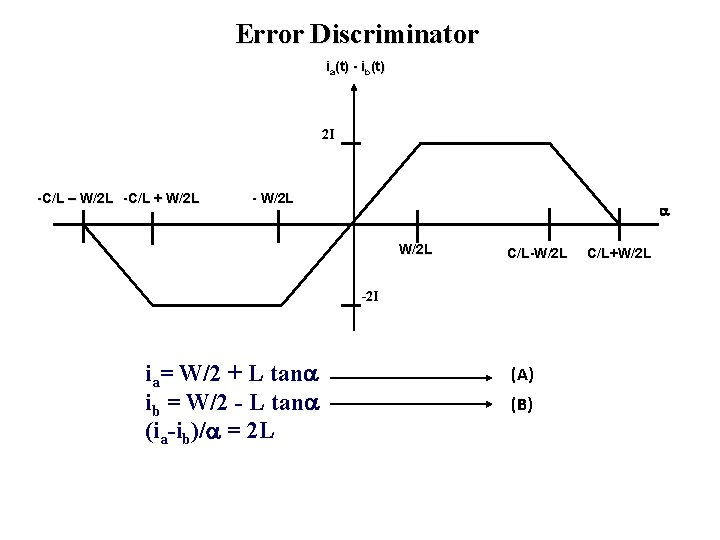Error Discriminator ia(t) - ib(t) 2 I -C/L – W/2 L -C/L + W/2 L - W/2 L C/L-W/2 L -2 I ia= W/2 + L tan ib = W/2 - L tan (ia-ib)/ = 2 L (A) (B) C/L+W/2 L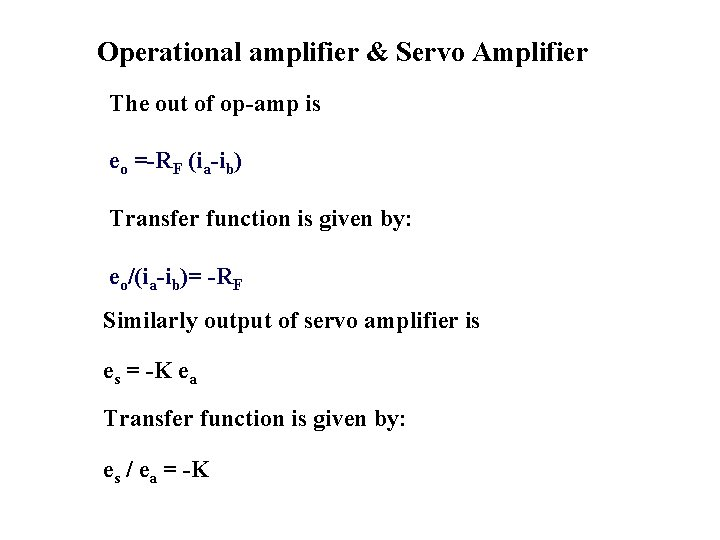Operational amplifier & Servo Amplifier The out of op-amp is eo =-RF (ia-ib) Transfer function is given by: eo/(ia-ib)= -RF Similarly output of servo amplifier is es = -K ea Transfer function is given by: es / ea = -K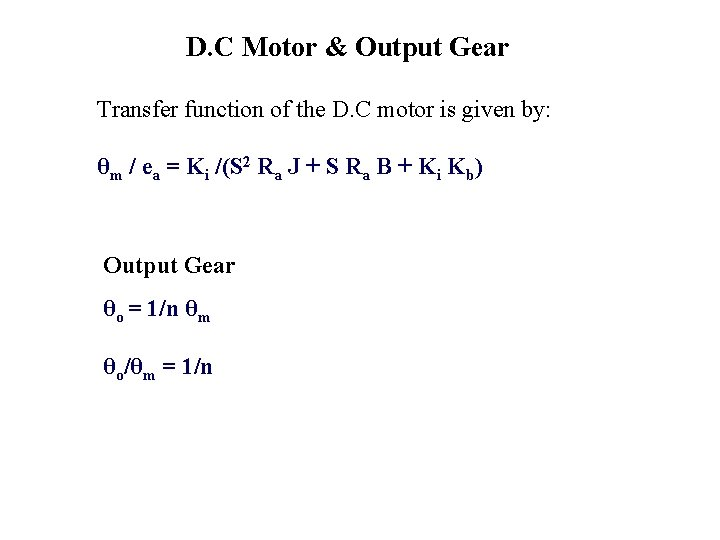D. C Motor & Output Gear Transfer function of the D. C motor is given by: θm / ea = Ki /(S 2 Ra J + S Ra B + Ki Kb) Output Gear θo = 1/n θm θo/θm = 1/n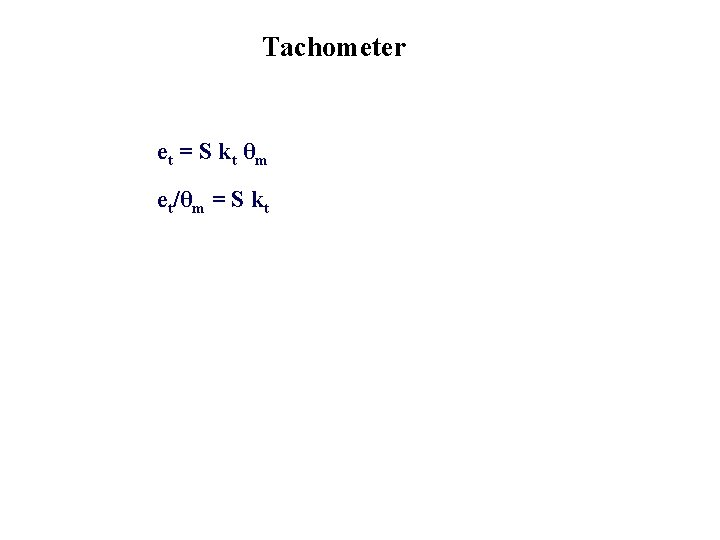Tachometer et = S k t θm et/θm = S kt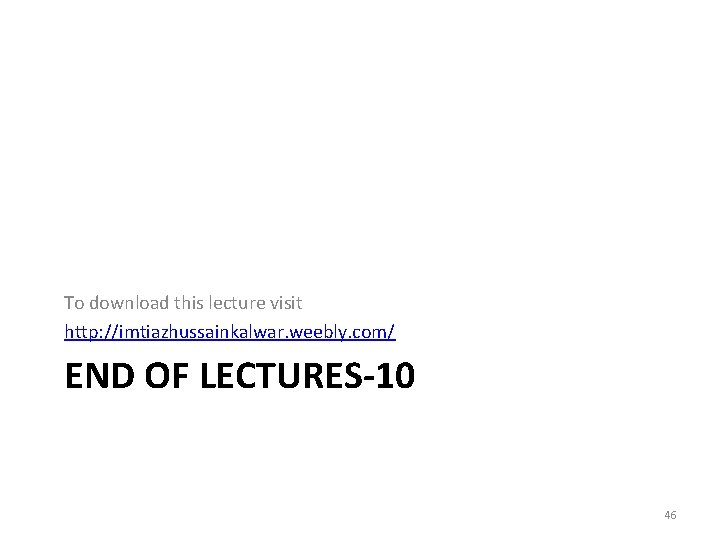To download this lecture visit http: //imtiazhussainkalwar. weebly. com/ END OF LECTURES-10 46